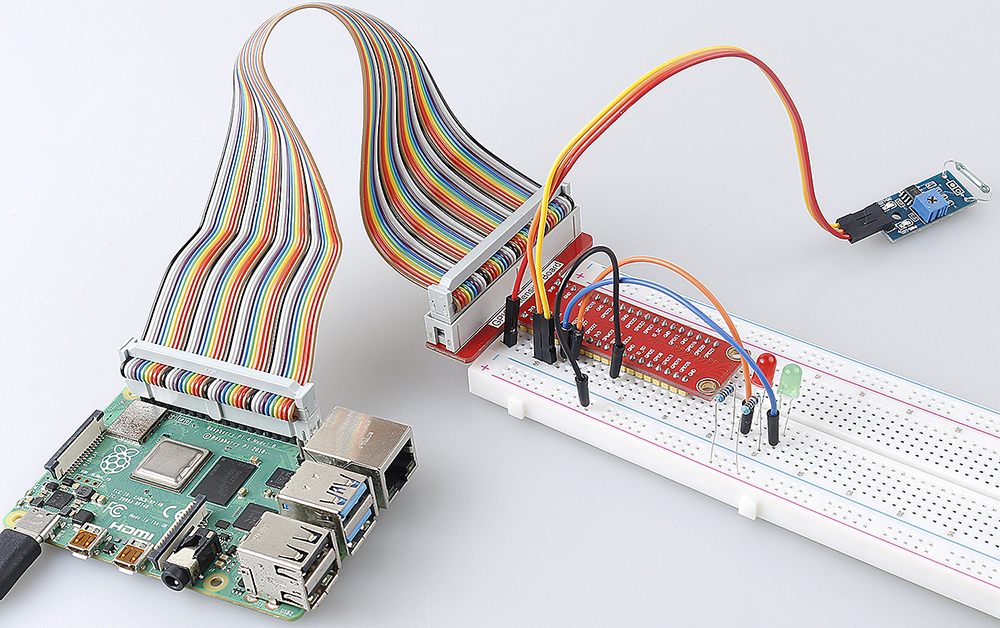# 2.2.4 Reed Switch Module¶

## Introduction¶

In this project, we will learn about the reed switch, which is an electrical switch that operates by means of an applied magnetic field.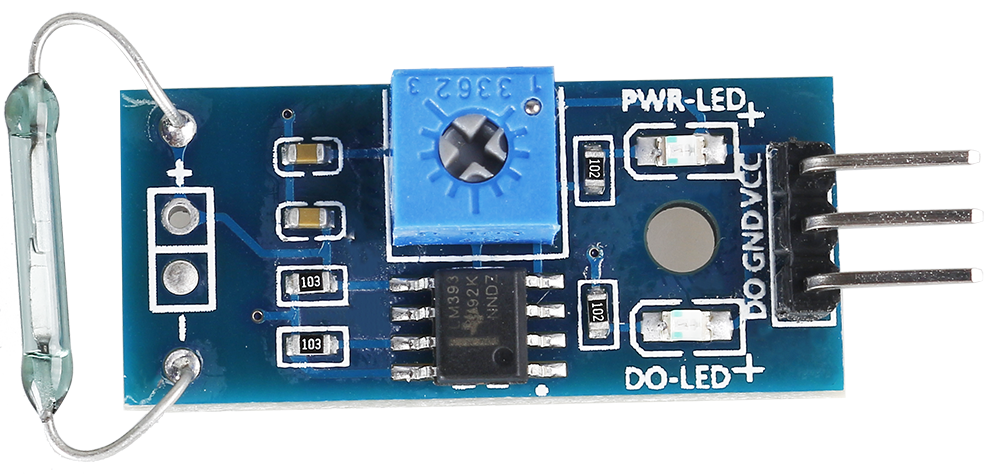## Required Components¶

In this project, we need the following components.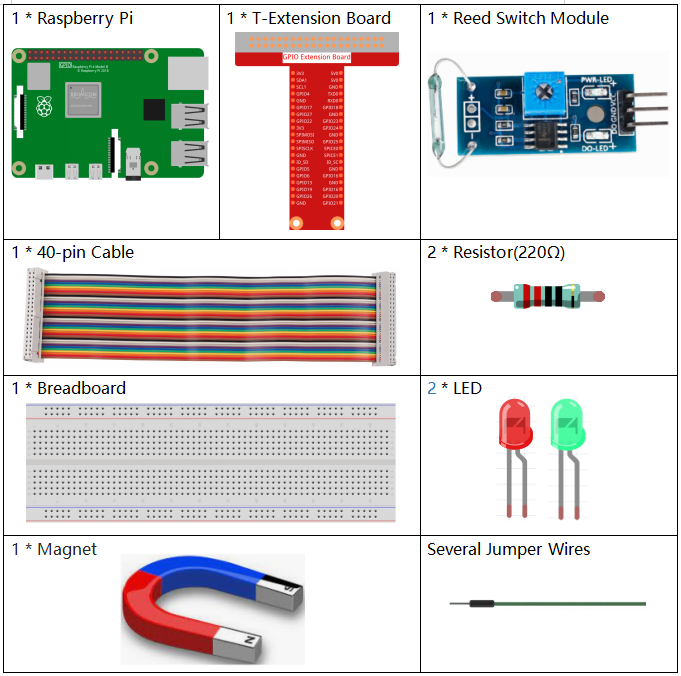Name

ITEMS IN THIS KIT

Raphael Kit

337

Raphael Kit

COMPONENT INTRODUCTION

GPIO Extension Board

Jumper Wires

Resistor

LED

Reed Switch Module

## Schematic Diagram¶

 T-Board Name physical wiringPi BCM GPIO17 Pin 11 0 17 GPIO27 Pin 13 2 27 GPIO22 Pin 15 3 22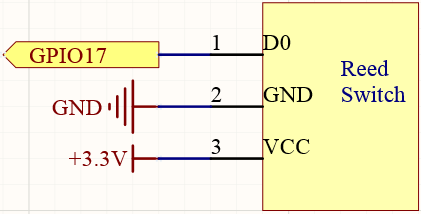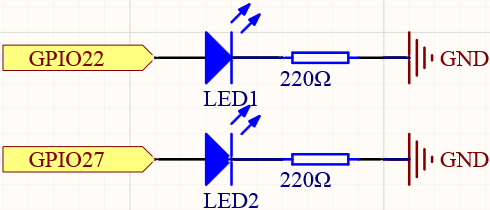## Experimental Procedures¶

Step 1: Build the circuit.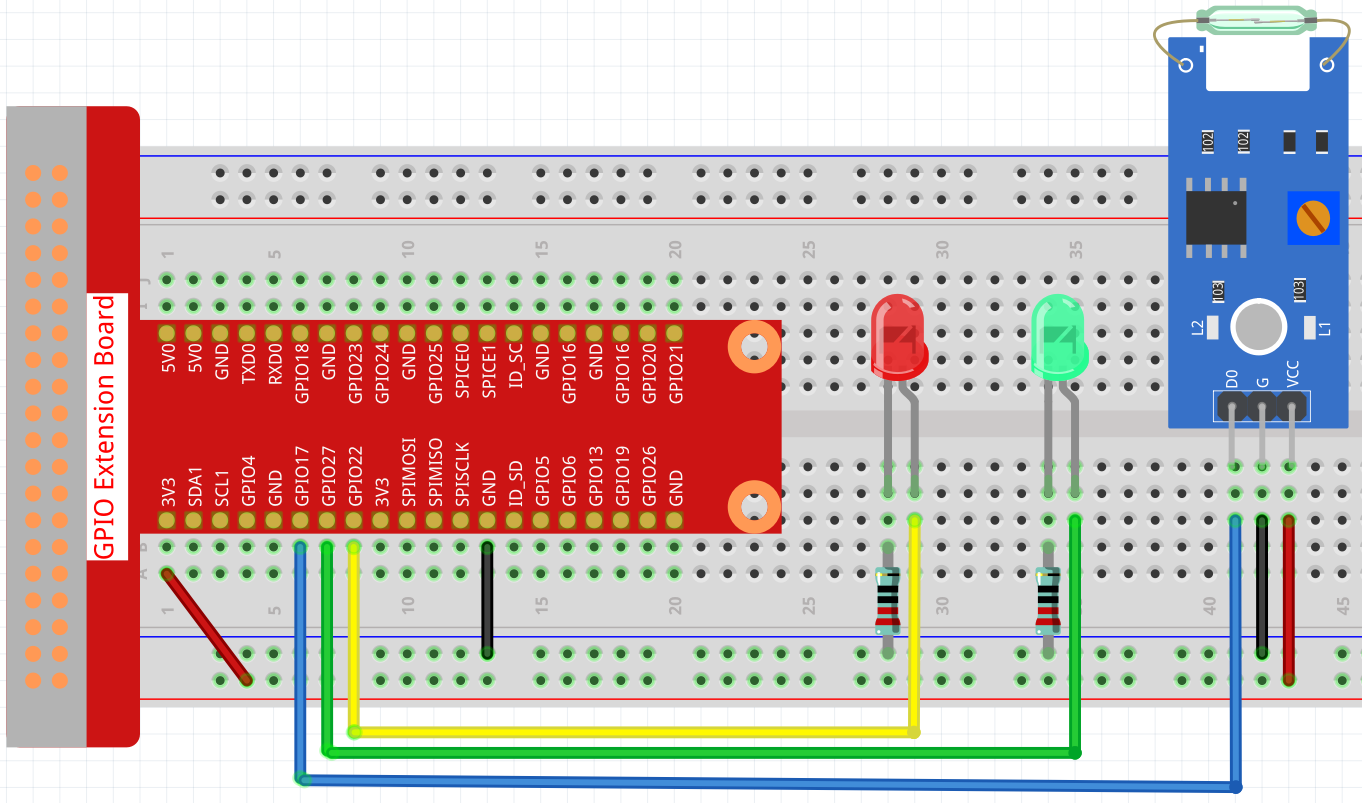Step 2: Change directory.

```cd ~/raphael-kit/python/
```

Step 3: Run.

```sudo python3 2.2.4_ReedSwitch.py
```

The green LED will light up when the code is run. If a magnet is placed close to the reed switch module, the red LED lights up; take away the magnet and the green LED lights up again.

Code

Note

You can Modify/Reset/Copy/Run/Stop the code below. But before that, you need to go to source code path like `raphael-kit/python`. After modifying the code, you can run it directly to see the effect.

```#!/usr/bin/env python3
import RPi.GPIO as GPIO
import time

ReedPin = 17
Gpin    = 27
Rpin    = 22

def setup():
GPIO.setmode(GPIO.BCM)       #
GPIO.setup(Gpin, GPIO.OUT)     # Set Green Led Pin mode to output
GPIO.setup(Rpin, GPIO.OUT)     # Set Red Led Pin mode to output
GPIO.setup(ReedPin, GPIO.IN, pull_up_down=GPIO.PUD_UP)    # Set ReedPin's mode is input, and pull up to high level(3.3V)

def Led(x):
if x == 0:
GPIO.output(Rpin, 1)
GPIO.output(Gpin, 0)
if x == 1:
GPIO.output(Rpin, 0)
GPIO.output(Gpin, 1)

def detect(self):
Led(GPIO.input(ReedPin))

def loop():
while True:
pass

def destroy():
GPIO.output(Gpin, GPIO.HIGH)       # Green led on
GPIO.output(Rpin, GPIO.LOW)       # Red led off
GPIO.cleanup()                     # Release resource

if __name__ == '__main__':     # Program start from here
setup()
detect()
try:
loop()
except KeyboardInterrupt:  # When 'Ctrl+C' is pressed, the child program destroy() will be  executed.
destroy()
```

Code Explanation

```ReedPin = 17
Gpin    = 27
Rpin    = 22

def setup():
GPIO.setmode(GPIO.BCM)       #
GPIO.setup(Gpin, GPIO.OUT)     # Set Green Led Pin mode to output
GPIO.setup(Rpin, GPIO.OUT)     # Set Red Led Pin mode to output
GPIO.setup(ReedPin, GPIO.IN, pull_up_down=GPIO.PUD_UP)    # Set ReedPin's mode is input, and pull up to high level(3.3V)
```

Set the GPIO modes to BCM Numbering. `ReedPin`, `Gpin` and `Rpin` connects to the GPIO17, GPIO27 and GPIO22.

`GPIO.add_event_detect()` is used to add an event that is triggered by a change in the value (level) of `ReedPin`, at which point the callback function `detect()` is called.

```def Led(x):
if x == 0:
GPIO.output(Rpin, 1)
GPIO.output(Gpin, 0)
if x == 1:
GPIO.output(Rpin, 0)
GPIO.output(Gpin, 1)
```

Define a function `Led()` to turn the two LEDs on or off. If `x=0`, the red LED lights up; otherwise, the green LED will be lit.

```def detect(self):
Led(GPIO.input(ReedPin))
```

In this callback function, the value of the reed switch is used to control the 2 LEDs.

## Phenomenon Picture¶